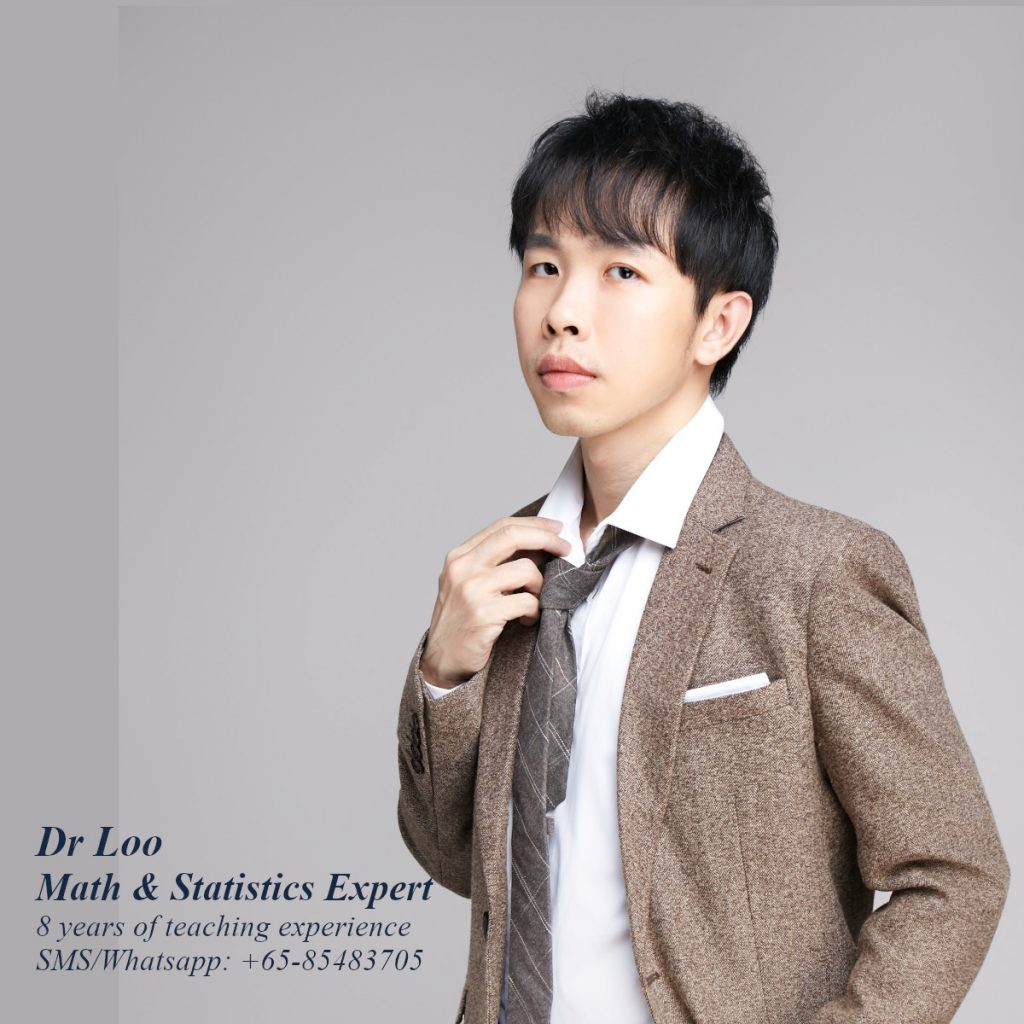+65-85483705
Select Page

# Logarithms

When learning logarithm function in secondary mathematics classes, students are likely to introduced with the law of logarithms and also their corresponding graph. In addition to that, they are also likely to deal with situation whereby they need to solve logarithmic functions. Sometime knowing on how to change the base of logarithms can be useful when solving the function.

Question

Solve the following questions.

1. 3x = 25
2. log3x = 2
3. log35

1. x = 1.3979/0.4771 = 2.929
2. 9
3. x = 0.69897/0.4771 = 1.465

Note:

• If you remember the laws of logarithms, you may notice that sometimes logarithms expression can be written in different ways.
• You may see from below on how the logarithm expression can be simplied (eg. when logorithms with same base be added or subtracted from one another).

### The laws of logarithms

• logam + logan = loga(m * n) = logamn
• logam – logan = loga(m / n)
• logamb = b*logam
• loga1 = 0
• logaa = 1
• alogax = x
• logax = logbx/logba

## About the Math Tutor - Dr Loo

I am a PhD holder with 9 years of teaching experience at secondary school and university. My expertizes are mathematics, statistics, econometrics, finance and machine learning.

Currently I do provide consultation and private tuition in Singapore. For those who are looking for math tutor in Singapore or statistics tutor in Singapore, please feel free to contact me at +65-85483705 (SMS/Whatsapp/Telegram).

Some of the math tuition in Singapore I provide includes O-level math tuition and also JC Math Tuition (H1 Math Tuition & H2 Math Tuition). On the other hand, my statistics tuition in Singapore mostly focus on pre-university level until postgraduate level. For those who prefer online tutoring service, the online O-level math tuition and online JC math tuition are also available.## For those who are looking for math tution in Singapore

Need help with this topic? I do provide mathematics home tuition in Singapore for O-level math and also JC H2 math. In addition, online math tutoring is available as well. Feel free to contact me if you would like to know further.